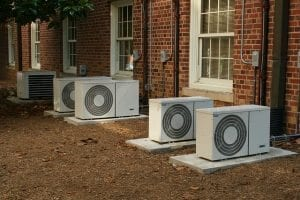## Electrical Formulas

E= voltage (emf)
I = amperage (current)
R = resistance (load)

### Ohm’s law states:

E = I x R which also means:
I = E/R
R= E/I

### Watts

P = E x I
or if your P is in kilowatts:
P = (E x I)/1000

### U Factor

U = 1/R That is, if R = 4, U = ¼, or 0.25

### 3 Phase Voltage Unbalance

= (100 x maximum deg. from average volts) / Average Volts

### Farads

One Farad capacity: 1 amp stored under 1 volt of pressure
MFD (microfarad) = 1 MFD = 1 Farad / 1,000,000

### Coulomb

= 6.24 X 1018 (1 Coulomb = 1 Amp)

VA (how the secondary transformer is rated) = volts x amps## Work and Horsepower

### Work

Work = force x distance
So if you weigh 180 lb, and climb stairs 100 ft high, the work is 180 lb x 100 ft = 18,000 ft-lbs (foot pounds)

### Horsepower (HP)

1 Horsepower = 33,000 ft-lb of work in 1 minute
HP to KW to BTU
1 HP = 746 Watts
1 KW =3413 BTU

## HVAC

### Ton of Refrigeration

The amount of heat to melt 1 ton of ice at 32 degrees F. This amount is 12,000 BTU per hour, which is 288,000 BTU in 24 hours

### RPM

RPM of motor = (60Hz x 120) / (No. of Poles)
1800 RPM Motor – slippage makes it about 1750
3600 RPM Motor – slippage makes it about 3450## What’s in the Air

Dry Air = 78.0% Nitrogen 21.0% Oxygen 1.0% Other Gases
Wet Air = Same as dry air plus water vapor
Specific Density = 1 / Specific Volume
Specific Density Of Air = 1 / 13.33 = .075 lbs./cu.ft.
Standard Air = 24 Specific Heat (BTU’s needed to raise 1 lb. 1 degree)

### Heat and Humidity

Enthalphy = h = Sensible heat + Latent heat
Relative Humidity = Moisture present / Moisture air can hold
Specific Humidity = Grains of moisture per dry air 7000 GRAINS in 1 lb. of water
Dew Point = When wet bulb equals dry bulb
Total Pressure (Ductwork) = Static Pressure + Velocity Pressure
28 Inches Of Wc (water column) = 1 psi
Furnace Efficiency: % Efficiency = energy output / energy input

## Useful HVAC Formulas

### For conditions other than standard air:

Total Heat (BTU/hr) = 4.5 x cfm x Δh (std. air)
Sensible Heat (BTU/hr) = 1.1 x cfm x Δt (std. air)
Latent Heat (BTU/hr) = 0.69 x cfm x Δgr. (std. air)

### Other Formulas

Total Heat (BTU/hr) = 500 x gpm x Δt (water)
GPM cooler = (24 x TONS) / Δt (water)
Fluid Mixture Tm = (Xt1 + Yt2) / X + Y (this works for air or water)
BTU/hr = 3.413 x watts = HP x 2546 = Kg Cal x 3.97
Lb. = 453.6 grams = 7000 grains
psi = ft. water/2.31 = in. hg/2.03 = in. water/27.7 = 0.145 x kPa
Ton = 12,000 BTU/hr = 0.2843 x KW
HP (air) = cfm x Δp (in.H2O)/6350 x Eff.
HP (water) = gpm x Δp (ft.)/3960 x Eff.
gpm = 15.85 x L/S
cfm = 2.119 x L/S
CFM = ______BTU/Hr / ( 1.08 x Temperature Difference)
Therm = 100,000 BTU = MJ/105.5
Watt/sq. ft. = 0.0926 x W/M²
PPM (by mass) = mg/kg

NOTE: the above information taken from "hvactraining101.com"